### Assessment of Models Based on Aggregates of Residuals

Lin, Wei, and Ying (2002) present graphical and numerical methods for model assessment based on the cumulative sums of residuals over certain coordinates (such as covariates or linear predictors) or some related aggregates of residuals. The distributions of these stochastic processes under the assumed model can be approximated by the distributions of certain zero-mean Gaussian processes whose realizations can be generated by simulation. Each observed residual pattern can then be compared, both graphically and numerically, with a number of realizations from the null distribution. Such comparisons enable you to assess objectively whether the observed residual pattern reflects anything beyond random fluctuation. These procedures are useful in determining appropriate functional forms of covariates and link function. You use the ASSESS|ASSESSMENT statement to perform this kind of model-checking with cumulative sums of residuals, moving sums of residuals, or LOESS smoothed residuals. See Example 42.8 and Example 42.9 for examples of model assessment.

Let the model for the mean be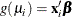where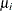is the mean of the responseand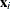is the vector of covariates for the ith observation. Denote the raw residual resulting from fitting the model as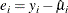and let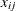be the value of the jth covariate in the model for observation i. Then to check the functional form of the jth covariate, consider the cumulative sum of residuals with respect to,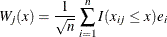where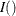is the indicator function. For any x,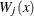is the sum of the residuals with values of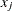less than or equal to x.

Denote the score, or gradient vector, by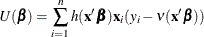where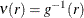, and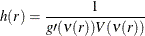Let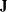be the Fisher information matrix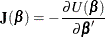Define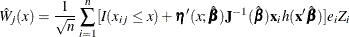where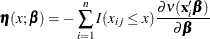and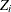are independent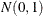random variables. Then the conditional distribution of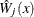, given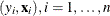, under the null hypothesisthat the model for the mean is correct, is the same asymptotically as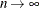as the unconditional distribution of(Lin, Wei, and Ying, 2002).

You can approximate realizations from the null hypothesis distribution ofby repeatedly generating normal samples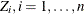, while holding, at their observed values and computingfor each sample.

You can assess the functional form of covariate j by plotting a few realizations ofon the same plot as the observedand visually comparing to see how typical the observedis of the null distribution samples.

You can supplement the graphical inspection method with a Kolmogorov-type supremum test. Let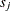be the observed value of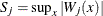. The p-value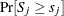is approximated by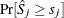, where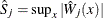.is estimated by generating realizations of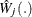(1,000 is the default number of realizations).

You can check the link function instead of the jth covariate by using values of the linear predictor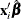in place of values of the jth covariate. The graphical and numerical methods described previously are then sensitive to inadequacies in the link function.

An alternative aggregate of residuals is the moving sum statistic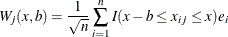If you specify the keyword WINDOW(b), then the moving sum statistic with window size b is used instead of the cumulative sum of residuals, with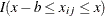replacing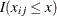in the earlier equation.

If you specify the keyword LOESS(f), loess smoothed residuals are used in the preceding formulas, where f is the fraction of the data to be used at a given point. If f is not specified,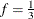is used. For data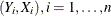, define r as the nearest integer to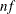and h as the rth smallest among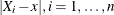. Let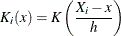where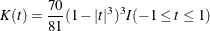Define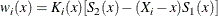where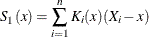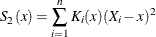Then the loess estimate of Y at x is defined by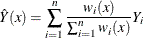Loess smoothed residuals for checking the functional form of the jth covariate are defined by replacing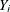with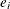and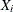with. To implement the graphical and numerical assessment methods,is replaced with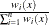in the formulas forand.

You can perform the model checking described earlier for marginal models for dependent responses fit by generalized estimating equations (GEEs). Let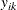denote the kth measurement on the ith cluster,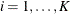,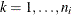, and let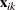denote the corresponding vector of covariates. The marginal mean of the response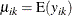is assumed to depend on the covariate vector by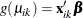where g is the link function.

Define the vector of residuals for the ith cluster as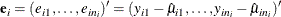You use the following extension ofdefined earlier to check the functional form of the jth covariate: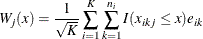where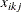is the jth component of.

The null distribution ofcan be approximated by the conditional distribution of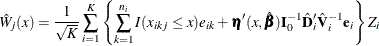where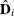and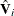are defined as in the section Generalized Estimating Equations with the unknown parameters replaced by their estimated values,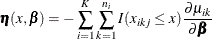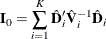and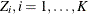, are independentrandom variables. You replacewith the linear predictor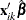in the preceding formulas to check the link function.